o
osc_a22drz29

# 1. 线性模型简介

## 0x2：线性模型的基本形式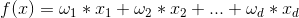f(x)为离散的值：线性多分类模型
f(x)为实数域上实值函数：线性回归模型
f(x)为对数：对数线性模式
f(x)进行sigmoid非线性变换：对数几率回归
...

### 1. 线性模型参数求解的本质 - 线性方程组求解

y1 = 1 * w1 + 2 * w2 + .... + 3 * wn；
....
yn = 3 * w1 + 4 * w2 + .... + 3 * wn；

## 0x3：线性模型蕴含的基本思想

1. 原子可叠加性：许多功能更为强大的非线性模型（nonlinear model）可在线性模型的基础上通过引入层级结构或高维映射而得到；
2. 可解释性（comprehensibility）：权重向量 w 直观表达了各个属性在预测中的重要性（主要矛盾和次要矛盾），而误差偏置 b 则表达了从物理世界到数据表达中存在的不确定性，即数据不能完整映射物理世界中的所有隐状态，一定存在某些噪声无法通过数据表征出来；

https://www.cnblogs.com/jasonfreak/p/5551544.html
https://www.cnblogs.com/jasonfreak/p/5554407.html
http://www.cnblogs.com/jasonfreak/p/5595074.html
https://www.cnblogs.com/pengyingzhi/p/5383801.html

# 2. 线性回归 - 基于线性模型的一种回归预测模型

## 0x1：线性回归模型的基本形式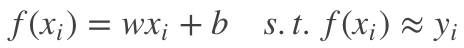，以尽可能准确地预测实值输出标记。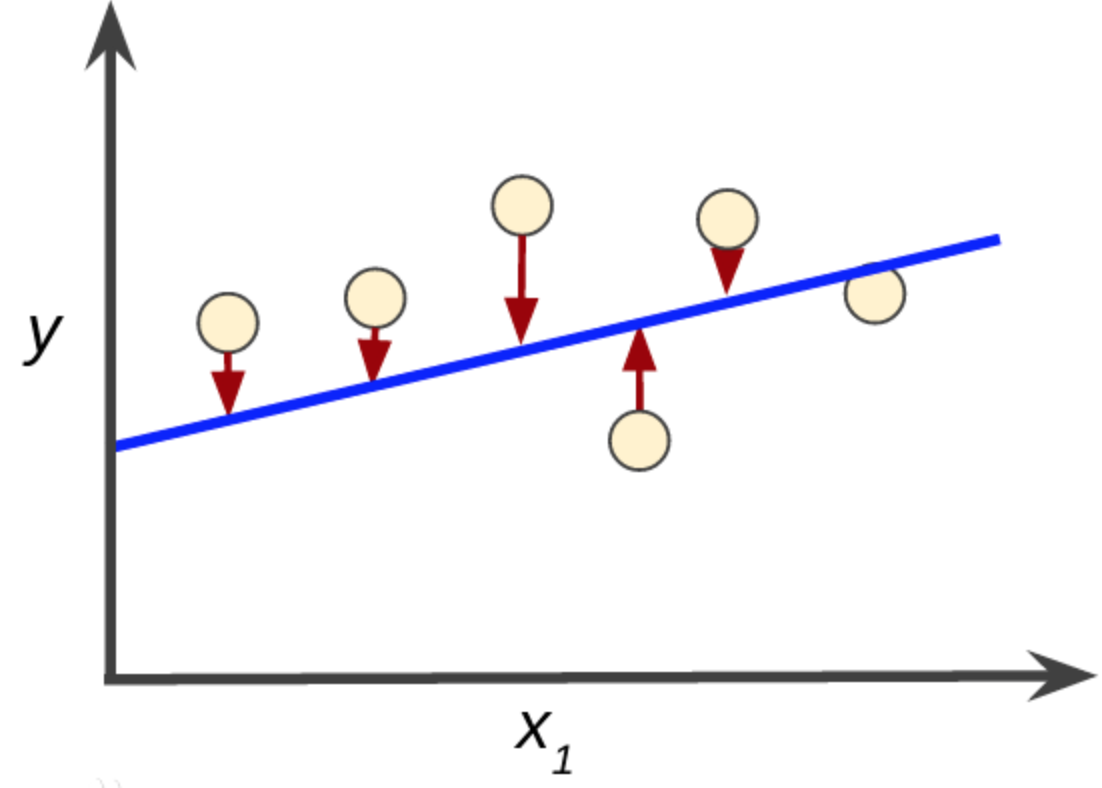## 0x2：线性回归模型中损失函数的选择

### 1. 最小二乘损失函数

#### 1）线性最小二乘的基本公式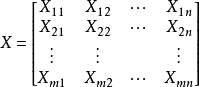，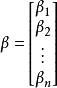，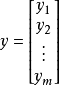#### 2）为什么是最小二乘？不是最小三乘或者四乘呢？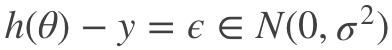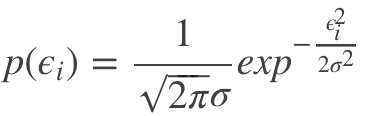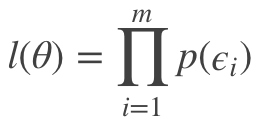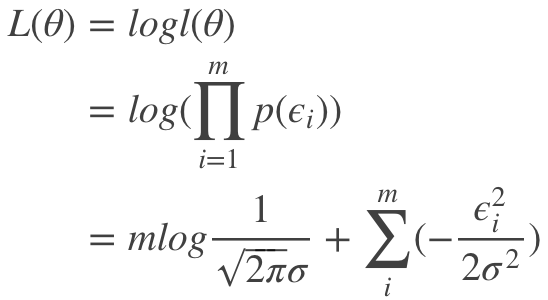#### 3）最小二乘参数求解方法

##### 3.1）偏导数为零求极值方法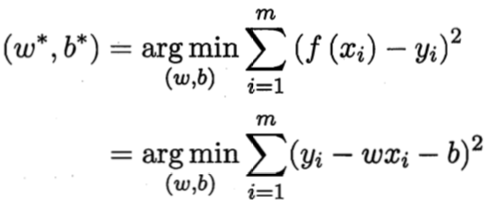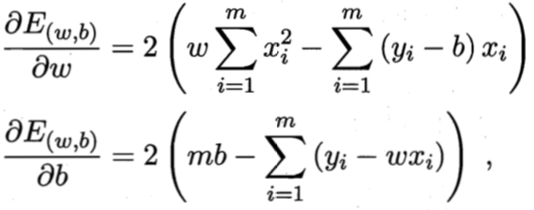https://www.zhihu.com/question/20822481
https://www.jianshu.com/p/985aff037938
https://juejin.im/entry/5be53a575188257cf9715723 
##### 3.2）逆矩阵计算参数求解方法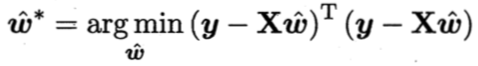令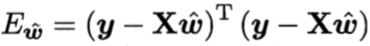，对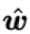求导得到：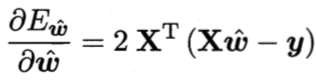1）满秩情况下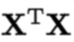为满秩矩阵（full-rank matrix）或正定矩阵（positive definite matrix）时，令上面求导公式为零可得：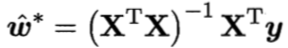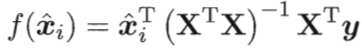2）非满秩情况下

https://zhuanlan.zhihu.com/p/34842727
https://zhuanlan.zhihu.com/p/33899560
https://blog.csdn.net/shiyongraow/article/details/77587045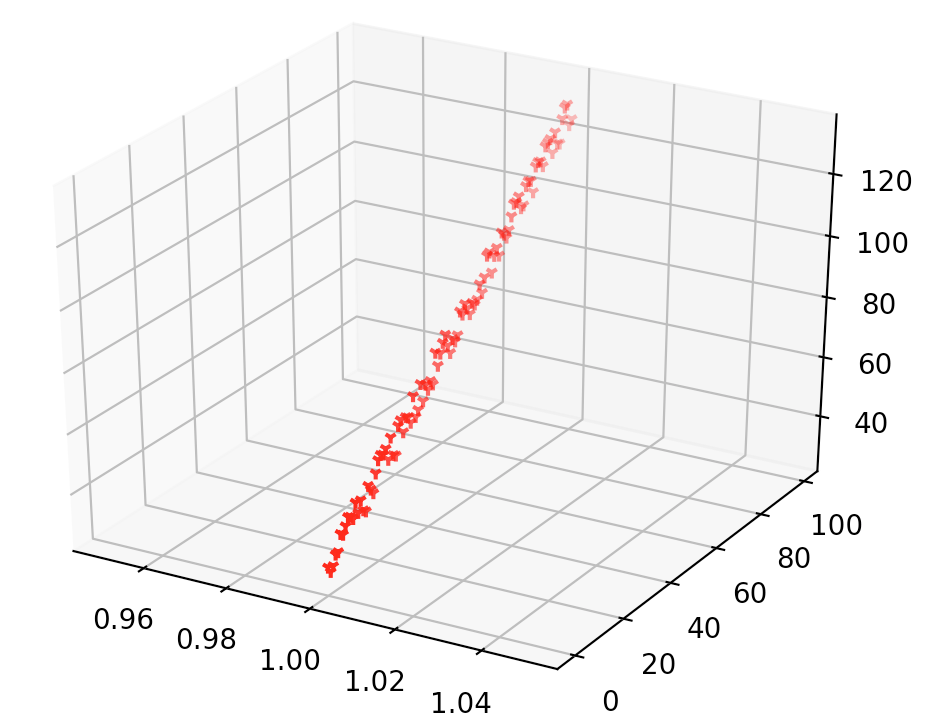# -*- coding: utf-8 -*-

import matplotlib.pyplot as plt
from mpl_toolkits.mplot3d import Axes3D import numpy as np import random from sklearn import linear_model #首先要生成一系列数据，三个参数分别是要生成数据的样本数，数据的偏差，以及数据的方差 def getdata(samples, bias, variance): X = np.zeros(shape=(samples, 2)) #初始化X Y = np.zeros(shape=samples) for i in range(samples): X[i] = 2 * i X[i] = i Y[i] = (i + bias) + random.uniform(0, 1) * variance return X, Y # 梯度下降算法来解决最优化问题，求损失函数的最小值 def gradient(X, Y, alpha, m, iter_numbers): theta = np.ones(2) # 初始化theta的值 初始化要求解模型中参数的值 bias = np.ones(1) X_trans=X.transpose() # 转化为列向量 for i in range(iter_numbers): Y_hat = np.dot(X, theta) + bias # 待预测的函数: y = wx + b loss = Y_hat - Y # 得到的仍然是一个m*1的列向量 coss = np.sum(loss**2) / (2 * m) # 平方和损失函数 print("iteration:%d / Cost:%f"%(i, coss)) #打印出每一次迭代的损失函数值，正常情况下得到的损失函数随着迭代次数的增加该损失函数值会逐渐减少 gradient_theta = np.dot(X_trans, loss) / m #损失函数的对 theta 参数求导后的结果，后面梯度下降算法更新参数theta的值时会用到这一项的值 gradient_bias = np.sum(loss) / m #损失函数的对 b 参数求导后的结果 theta = theta - alpha * gradient_theta bias = bias - alpha * gradient_bias return theta, bias if __name__=="__main__": m = 100 X, Y = getdata(m, 25, 10) # Plot data ax = plt.subplot(111, projection='3d') ax.scatter(X[:,0], X[:,1], Y, c='r',marker='1') theta, bias = gradient(X, Y, 0.00002, m, 2000000) print("GD -> the parameters (theta) is: ", theta) print("GD -> the parameters (bias) is: ", bias) # 打印GD得到的参数对应的函数曲线 X_GD = np.zeros(shape=(m, 2)) Y_GD = np.zeros(shape=m) for i in range(m): X_GD[i] = 2 * i X_GD[i] = i Y_GD[i] = theta * X_GD[i] + theta * X_GD[i] + bias ax.plot(X_GD[:,0], X_GD[:,1], Y_GD, c='b', lw=1) clf = linear_model.LinearRegression() clf.fit(X,Y) print("LSM -> the theta is: ", clf.coef_) print("LSM -> the bias is: ", clf.intercept_) # 打印MSE得到的参数对应的函数曲线 X_LSM = np.zeros(shape=(m, 2)) Y_LSM = np.zeros(shape=m) for i in range(m): X_LSM[i] = 2 * i X_LSM[i] = i Y_LSM[i] = theta * X_LSM[i] + theta * X_LSM[i] + bias ax.plot(X_LSM[:,0], X_LSM[:,1], Y_LSM, c='g') plt.show()

('GD -> the parameters (theta) is: ', array([0.2020337 , 0.60101685])) ('GD -> the parameters (bias) is: ', array([29.915184]))  ('LSM -> the theta is: ', array([0.40202688, 0.20101344])) ('LSM -> the bias is: ', 29.916313760692574)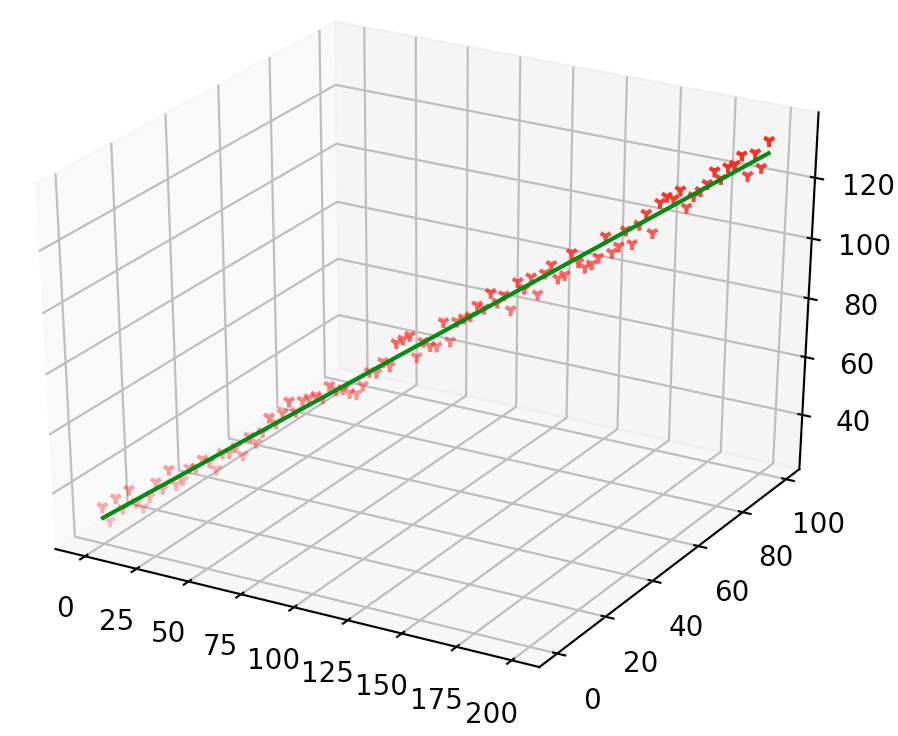1. 尽管使用了相同的损失函数，但是GD算法得到的结果和LSM最小二乘法的最优结果并不一致，GD算法只是在逼近最优值，且接近最优值，并不能在有限步骤内完全达到最优点；
2. GD算法是一种参数求解算法，和使用什么损失函数没有关系，笔者在代码中使用了MSE平方和损失误差，读者朋友可以自己换成交叉熵代价损失，并不影响最终结果。
3. 在梯度下降中，权值更新是一个迭代的过程，每个维度权值（wi）更新取决于当前轮次中的误差，误差较大大的个体（xi）会使得对应的 wi 调整的更剧烈，这点从代码中可以体现。这种权值更新办法比较直观，但是同时也比较低效：即人人都有发言的权利，每次只考虑部分人，容易顾此失彼。 相比之下，LSM直接基于大数定理进行最小化均方误差，本质上就是求每个属性维度 xi 的样本均值。
##### 3.4）其他参数优化算法

https://blog.csdn.net/Wang_Da_Yang/article/details/78594309

#### 4）最小二乘损失的几何意义

##### 3.1）三维空间的向量在二维平面的投影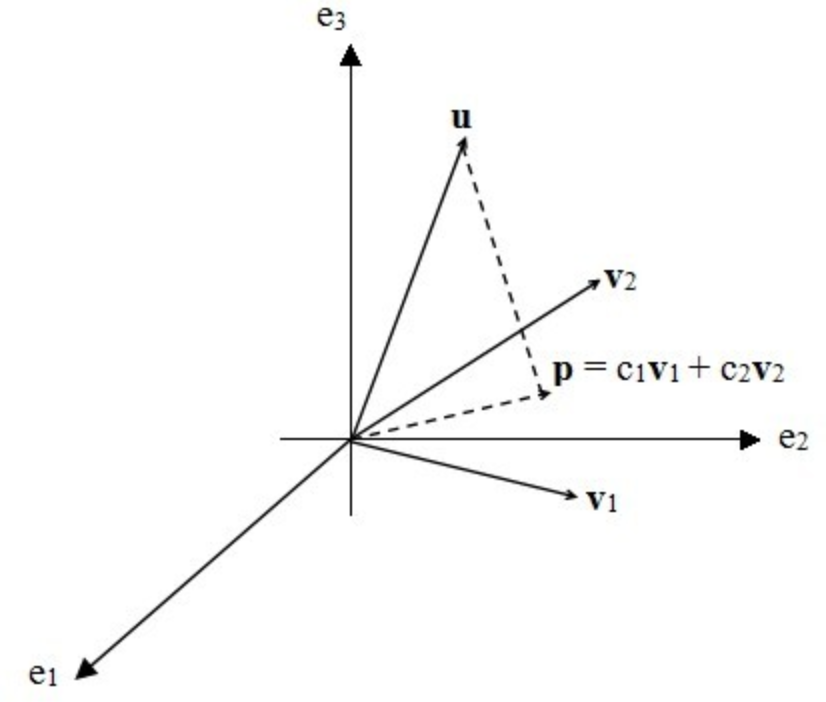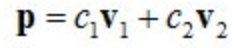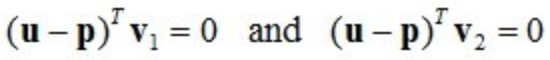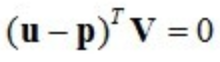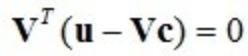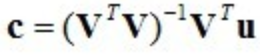##### 3.2）最小二乘和投影的关系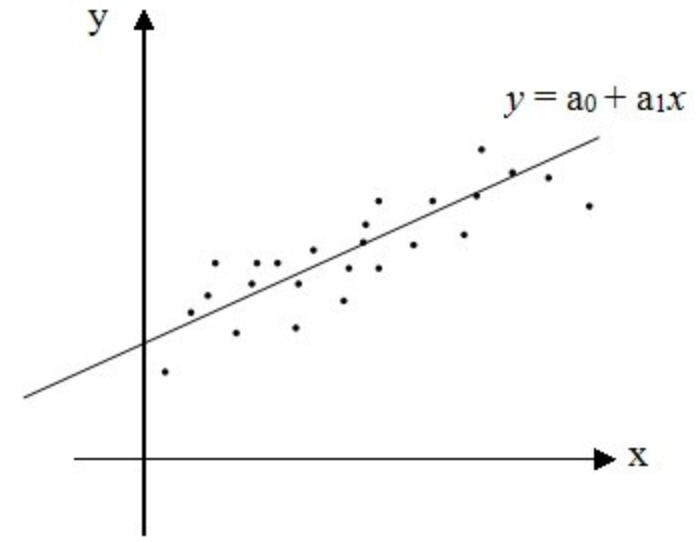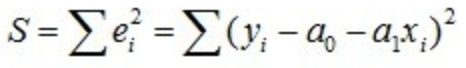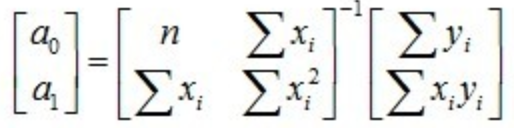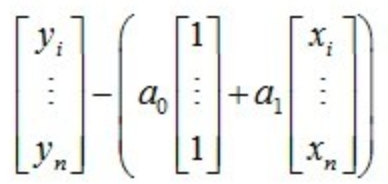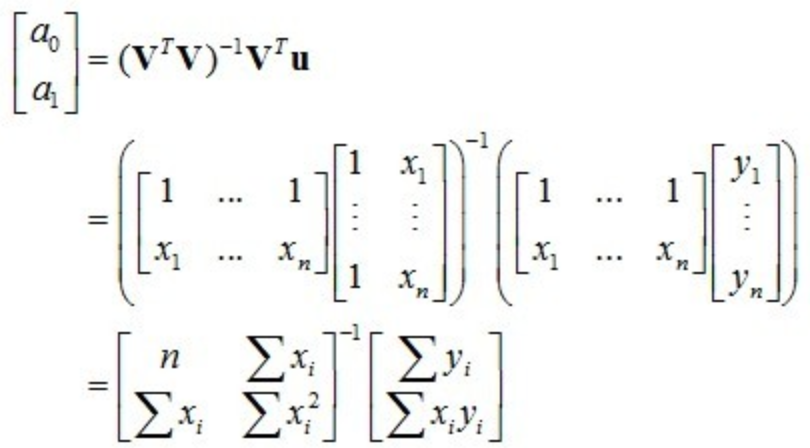https://www.jianshu.com/p/b49f28b1b98c
《线性代数的几何意义》西安电子科技大学出版社
https://blog.csdn.net/xiaoshengforever/article/details/14546075

### 2. 交叉熵代价函数

#### 1）熵(Entropy) - 表示一个事件A的自信息量

• 越不可能发生的事件信息量越大，比如"上帝存在"这句话信息量就很大。而确定事件的信息量就很低，比如"我是我妈生的"，信息量就很低甚至为0

• 独立事件的信息量可叠加。比如"a. 张三今天喝了阿萨姆红茶，b. 李四前天喝了英式早茶"的信息量就应该恰好等于a+b的信息量，如果张三李四喝什么茶是两个独立事件。

#### 3）KL散度的数学定义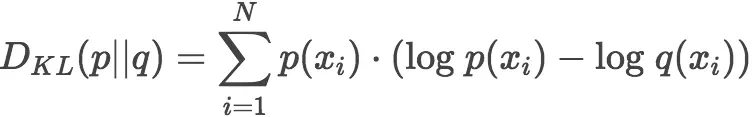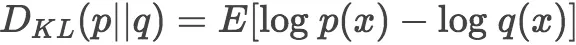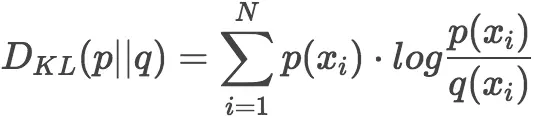#### 4）k-l散度的数学特性

• 如果 P（p） = P（q），即两个事件分布完全相同，那么KL散度等于0，当事件A为100%确定性事件时，分子为1，而当事件 q 越接近100%确定（即越靠近事件 p）时，总体KL散度公式趋向于0。
• KL散度公式中，减号左边的和公式事件 p 的熵有关，这是一个非常重要的理解KL散度的视角
• KL散度是严格顺序的，如果颠倒一下顺序，那么结果就不一样了。所以KL散度来计算两个分布 p 与 q 的时候是不是对称的，有"坐标系"的问题。

#### 5）交叉熵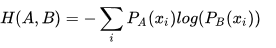• 和KL散度相同，交叉熵也不具备对称性：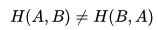；
• Cross(交叉)主要是用于描述这是两个事件之间的相互关系，对自己求交叉熵等于熵。即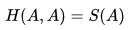#### 6）交叉熵在一定条件下等价于KL散度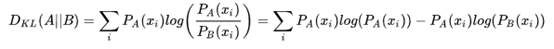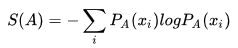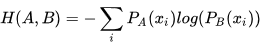#### 7）交叉熵作为损失函数在机器学习中的作用

https://zhuanlan.zhihu.com/p/39682125
https://www.cnblogs.com/kexinxin/p/9858573.html
https://blog.csdn.net/qq_17073497/article/details/81485650
https://blog.csdn.net/jiaowosiye/article/details/80786670
https://blog.csdn.net/u014135091/article/details/52027213
https://www.jianshu.com/p/3e163b6b96f5
https://blog.csdn.net/pxhdky/article/details/82388964
https://www.jianshu.com/p/43318a3dc715
https://www.zhihu.com/question/41252833  

# 3. 对数几率回归 - 基于线性回归的一种概率函数

## 0x1：阶跃函数 - 硬分类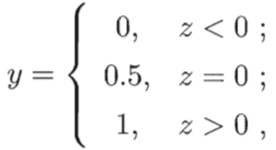## 0x2：sigmoid函数（对数几率函数） - 软分类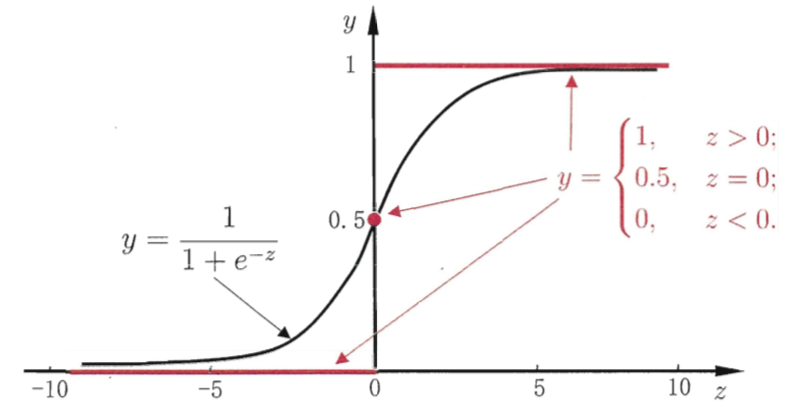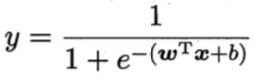=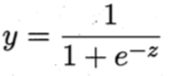### 1. logistic function中体现的几率性质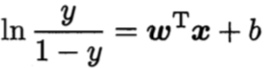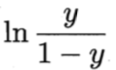## 0x3：对数几率回归的优点性质

1. 它直接对分类可能性进行建模，是一种判别式模型，无需事先假设数据分布，这样就避免了假设分布不准确所带来的问题；
2. 它不仅是预测出“类别”，而是可得到近似概率预测，这对需要需要利用概率辅助决策的任务很有用；
3. 对数几率函数是任意阶可导的凸函数，有很好的数学性质，现有的许多数值优化算法都可以直接用于求取最优解；

## 0x4：求解模型参数（w，b）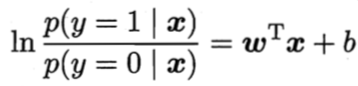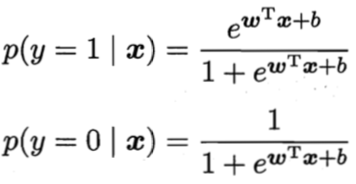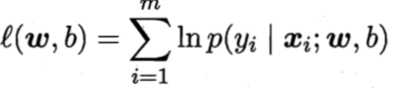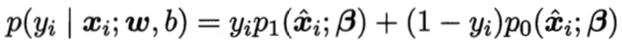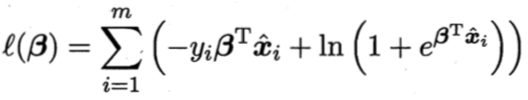，即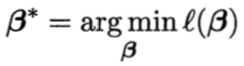https://baike.baidu.com/item/%E5%AF%B9%E6%95%B0%E5%87%A0%E7%8E%87%E5%9B%9E%E5%BD%92/23292667?fr=aladdin

# 4. 广义线性回归

## 0x1：对数线性回归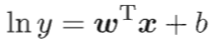。这就是“对数线性回归（log-linear regression）”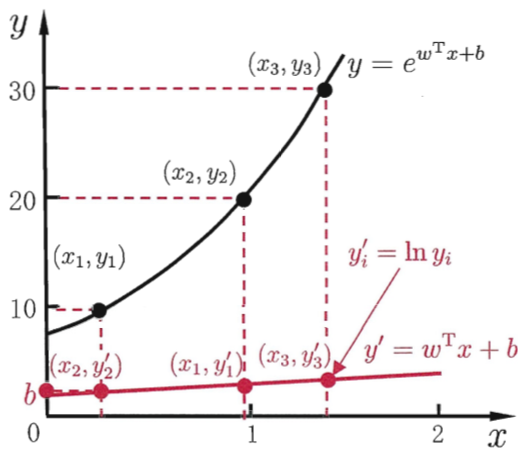## 0x2：广义线性模型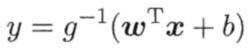https://baike.baidu.com/item/%E5%B9%BF%E4%B9%89%E7%BA%BF%E6%80%A7%E6%A8%A1%E5%9E%8B/8465894?fr=aladdin 

# 4. 线性判别分析（Fisher linear discriminant analysis） - 基于线性模型的线性投影判别算法

## 0x1：LDA的思想

LDA的思想非常朴素：

1. 给定训练样例集，设法将样例投影到一条直线上，使得同类样例的投影点尽可能接近、异类样例的投影点尽可能远离；
2. 在对新样本进行分类时，将其投影到同样的这条直线上，再根据投影点的位置来确定新样本的类别；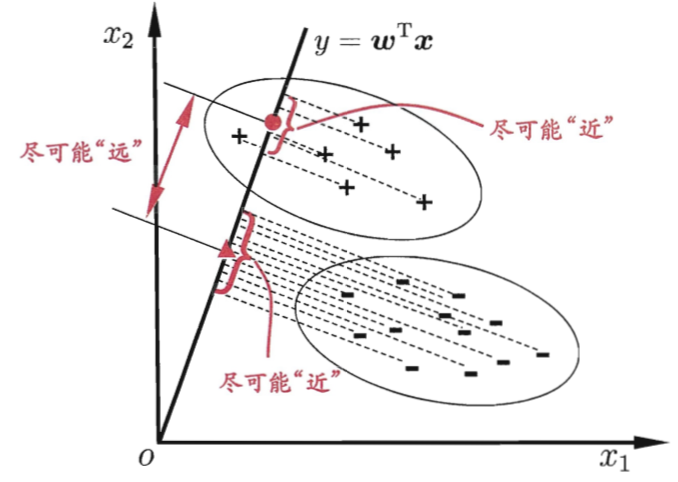## 0x3：LDA算法求最优解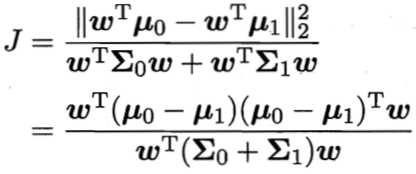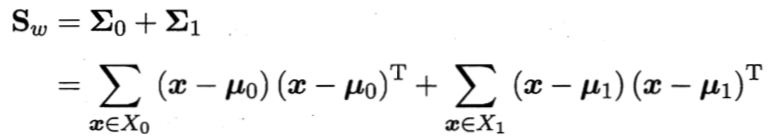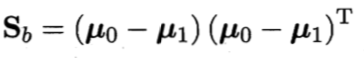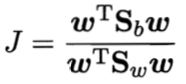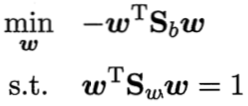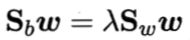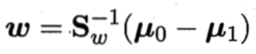## 0x4：LDA和PCA的内在共通之处

PCA是为了去除原始数据集中冗余的维度，让投影子空间的各个维度的方差尽可能大，也就是熵尽可能大；LDA是通过数据降维找到那些具有discriminative的维度，使得原始数据在这些维度上的投影，不同类别尽可能区分开来，而同类别之间尽量相近。

https://www.cnblogs.com/jiahuaking/p/3938541.html
https://www.cnblogs.com/LeftNotEasy/archive/2011/01/08/lda-and-pca-machine-learning.html 

# 5. 类别不平衡问题，及其缓解手段

## 0x2：类别不平衡带来的影响的原理分析

y 实际上表达了正例的可能性，几率 y/1-y 在反映了正例可能性和反例可能性的比值，阈值设置为0.5恰表明了分类器认为真实正、反例可能性相同，即分类器决策规则为：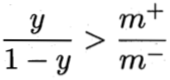：预测为正例.

gap = (1 - y/1-y) *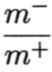## 0x3：类别不平衡问题的一种解决策略 - 再缩放（rescaling）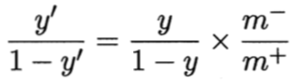## 0x4：类别不平衡问题的另一种解决策略 - 代价敏感学习（cost-sensitive learning）

cost+是将正例误分为反例的代价：如果更加关注正例别漏报了，就加大cost+惩罚比例；
cost-是将反例误分为正例的代价：如果更加关注反例别分错了，就加大cost-惩罚比例；

## 0x5：如何利用类别不平衡问题实现特定的分类策略

1. 训练集中，有意的将白样本：黑样本的比例设置的比较大，例如 5：1，甚至更大，人为的造成一个类别不平衡偏置，这么做的结果很容易理解，模型判黑的几率会降低，会更倾向于判白，也即降低了误报的几率；
2. 在训练中，进行自定义损失函数，keras/tensorflow中很容易做到这点，将“白判黑损失”认为的增加为“黑判白”的 N 倍，这么做的结果和第一点也是一样的；  

https://stackoverflow.com/questions/45961428/make-a-custom-loss-function-in-keras

o

### osc_a22drz29#### 暂无文章

26分钟前
7
0

50分钟前
5
0
jQuery Ajax错误处理，显示自定义异常消息 - jQuery Ajax error handling, show custom exception messages

56分钟前
28
0

51
0
spring源码解析-xml配置文件读取

wc_飞豆

22
0